### Get Prime Factors on the TI-85 and TI-86

This page demonstraes the use of the GETPRIME program on the TI-85 and TI-86. This program asks a user for a whole number and then the program determines the prime factorization of that number. The program produces a "nice looking" output. In order to do this the program needs to use another program called TOSTR. The listing of that program is given as
 Listing of the TOSTR program for the TI-85 and TI-86.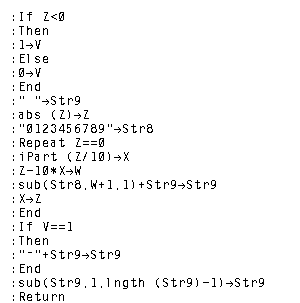To download, right-click on tostr.85p or tostr.86p.

The listing of the GETPRIME program follows:

 Part One of the GETPRIME listing for the TI-85 and TI-86 Part Two of the GETPRIME listing for the TI-85 and TI-86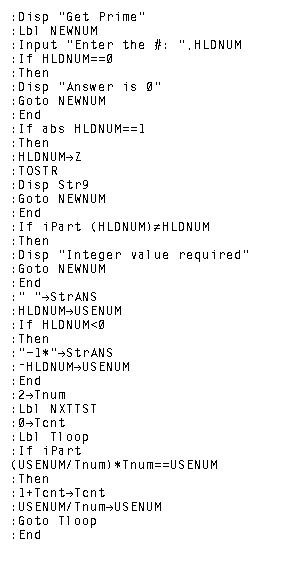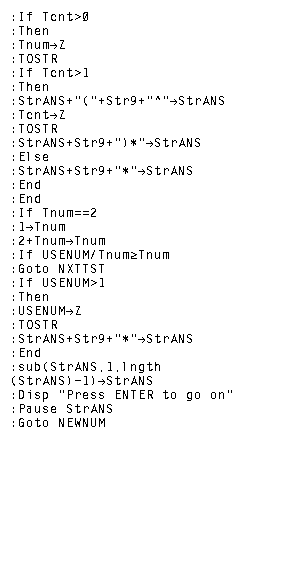To download the GETPRIME program for the TI-85 right-click on getprime.85p or to download the GETPRIME program for the TI-86 right-click on getprime.86p

The following images and discussions cover the use of the GETPRIME program on the TI-86. (The same images would appear on the TI-85.)

 Figure 1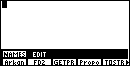In Figure 1 we have moved to the program menu via the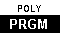key. On this particular calculator, the GETPRIME program is in the third position of that menu. Therefore, we can move to Figure 2 by pressing the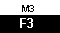key Figure 2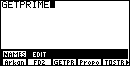The calculator has responded by pasting the command to run the selected program, in this case GETPRIME, onto the screen. We press the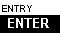key to tell the calculator to actually run the program. Figure 3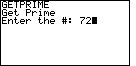The program starts by announcing its name and then by asking us for a number. In Figure 3 we have responded by entering the value 72 via the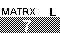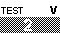key sequence. As usual, we press thekey to have the calculator accept our value and to start processing it. Figure 4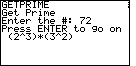The result of the program is shown in Figure 4. The calculator has factored 72 into (23)(32) which the calculator displays as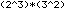At this point the calculator is in a paused condition; note the series of dots in the upper right corner of the calculator screen. We presskey to do another problem. Figure 5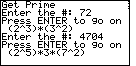In Figure 5 we ask the calculator to factor 4704, and the result is 25*3*72 Figure 6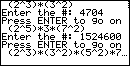Figure 6 demonstrates factoring 1524600. The result is 23*32*52*7*112 however, we can not see all of the answer in Figure 6. Because the calculator is in a paused condition after displaying the answer we can use the cursor keys to move the display to the right. Doing so will shift the display to that seen in Figure 7. Figure 7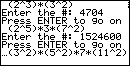Here we can see the rest of the answer started in Figure 6. Having seen the full answer we can press thekey again to move to a new problem. Figure 8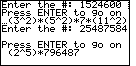Figure 8 shows the process of factoring 25487584. It is important to point out that it will take the calculator a while to produce that answer. An examination of the algorithm given above shows that the calculator will have to test all of the odd numbers between 5 and the square root of 796,487. Thus, the calculator tries to find a new factor by trying the odd numbers between 5 and 892. It will take the calculator a while to do this. One last issue is that of stopping the program. In this case we can break out of the program by pressing the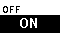key. The result is given in Figure 9. Figure 9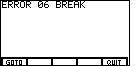In response to pressing the "ON" key, the calculator gives us the option of "Quiting" the program or "Going To" the program editor and, more specifically, to the line in the program that was being performed when we hit the "ON" key. Our interest is to quit the program. Therefore, we press the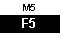key to select that option. Figure 10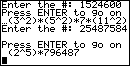Figure 10 shows the calculator having returned to normal mode. Note that the results of the program are still on the screen, but that the program is no longer running.